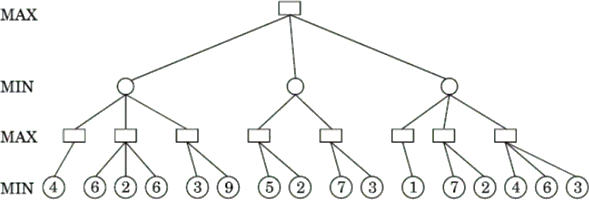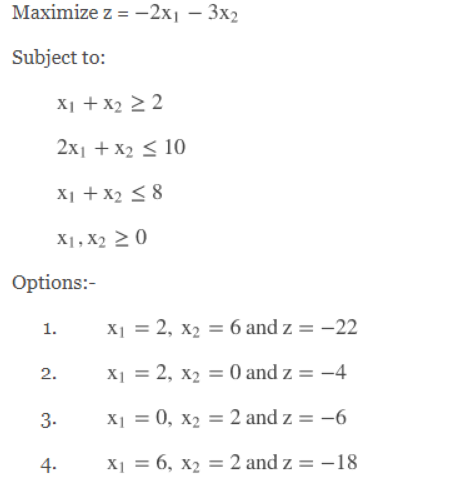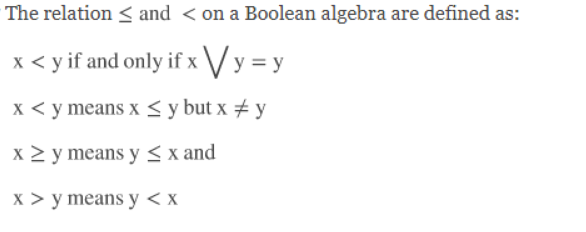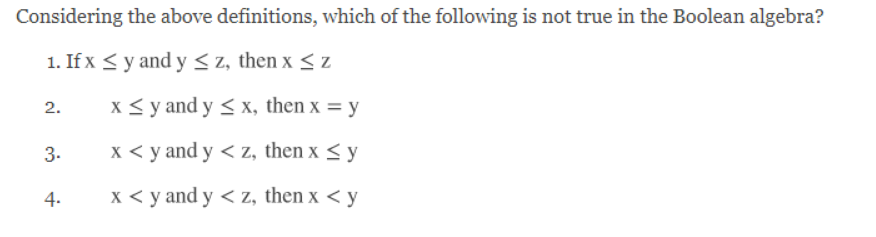A directory of Objective Type Questions covering all the Computer Science subjects. Here you can access and discuss Multiple choice questions and answers for various compitative exams and interviews.

#### Important Notice!

Dear users, compscibits.com is now permanently moved to compsciedu.com. Please update all your links and bookmarks accordingly. Soon, the site will be accessible through URL compsciedu.com only.

 131. Let A and B be two arbitrary events, then: a. P(A∩B) = P(A)P(B) b. P(AUB) = P(A)+P(B) c. P(AUB) ≤ P(A)+P(B) d. P(A/B) = P(A∩B)+P(B)

 132. The transitive closure of a relation R on set A whose relation matrixa. A b. B c. C d. D

 133. Consider the relation on the set of non-negative integers defined by x≡y if and only if: a. x mod 3=3 mod y b. 3 mod x≡3 mod y c. x mod 3=y mod 3 d. None of the above

 134. Minimum number of individual shoes to be picked up from a dark room (containing 10 pair of shoes) if we have to get atleast one proper pair: a. 2 b. 20 c. 11 d. None of these

 135. If f(x) =x+1 and g(x)=x+3 then fofofof is: a. g b. g+1 c. g^4 d. None of the above

 136. If Im denotes the set of integers modulo m, then the following are fields with respect to the operations of addition modulo m and multiplication modulo m:(i) Z23      (ii) Z29(iii) Z31    (iv) Z33 a. (i) only b. (i) and (ii) only c. (i), (ii) and (iii) only d. (i), (ii), (iii) and (iv)

 137. Consider the following minimax game tree search. What will be the value propagated at the root?a. 6 b. 3 c. 4 d. 5
 138. Use Dual Simplex Method to solve the following problem:a. 1 b. 2 c. 3 d. 4
 139. Choose the correct answer from the options given below:a. (iii) only b. (ii) and (iii) only c. (i) and (ii) only d. (iv) only